amortization.com Ltd.
Burlington, Ontario

905-639-0374
905-407-7988

info@amortization.comEffective Rates

The Effective Interest Rate (EIR) is an informative number. The EIR allows mortgages and loans to be compared before delving into points and transaction fees. If two loans or mortgages have the same EIR, they are equivalent and cost the borrower the same in interest. Using the EIR to compare loans or mortgages removes the uncertainty of not knowing the type of "compounding" used in the calculation of the interest factor. The APR on its own will not tell you what type of compounding was used.

Monthly payment mortgages using the 365 day year, exact day monthly interest factor method, have a slightly higher effective interest rate (EIR) as compared to the Bankers year monthly payment schedules that utilizes a 360 day year. With weekly and biweekly payment mortgages the opposite is true.

The MORTGAGE2 PRO CALCULATOR functions just like any other financial calculator. It uses the implied 360 day year because of the algebraic formulas. The only exception, is that the Days Per Year in the CALCULATOR only have an impact on the SPREADSHEET even though it is located in the CALCULATOR section. The effective interest rate (EIR) displayed in the CALCULATOR is for the 360 day year mode only. The EIR will not change when you change the days per year from 360 to 365.

360 day year weekly

In the calculator the effective interest rate is displayed to the right of the annual interest rate, 6% and 6.1677% respectively. A technique for determining what the weekly payments will accumulate to, is to to make all the SPREADSHEET payments zero for 20 years. Note, that we must use the same weekly payments of \$247.19 for 1040 weeks. After 1040 weeks the accumulation is \$496,530.67 Using the PV/FV Calculator window, the calculated Interest Rate per Period (because the Period is one year the number is the effective interest rate) is 16.167781% the same as the EIR in the CALCULATOR.

(Screenshot 1)

365 day year weekly

Now lets do the same example but change the days per year to 365. The PV/FV Calculator window, shows the calculated Interest Rate per Period to be 6.150374% which slightly less than 6.167781%

The effective interest rate for 365 day weekly and biweekly payment mortgages is slightly less than for a comparable weekly or biweekly utilizing a 360 day year.

(Screenshot 2)

Without getting into the algebraic formulas it is easy to show that this must be true merely by comparing the first line of a 360 day weekly schedule vs the first line of a 365 day weekly schedule, both for \$100,000. The 365 day schedule has less interest on the very first line, 114.85 vs 115.16 proof positive!!!

(Screenshot 3)
(Screenshot 4)

 Effective Interest Rate (EIR)

Below is an example of a negative amortization schedule (setting all the payments in the SPREADSHEET equal to zero) that shows what a cash flow of \$1,028.61 will accumulate to in 30 years using an annual interest rate of 12% which is equivalent to an effective interest rate (EIR), of 12.6825% The Principal box in the CALCULATOR is really the Present Value and some calculators label it that way.

(Screenshot 5)

If the compounding is Annual then annual interest rate and the effective interest rate are identical as can be readily seen below.

(Screenshot 6)

Rarely are loans and mortgages calculated annually. Knowing the EIR is important for many reasons.

1. There is no need to be concerned about the compounding method.

2. As a lender the EIR allows you to easily and quickly calculate your yield on a loan at the end of the year. A \$100,000 loan with an EIR of 12.6825% gives the lender a return of \$100,000 x 1.126825 = \$112, 682.50. The EIR is required in order to calculate the Future Value of a series of payments using a financial calculator.

If you borrow \$100,000 at 6% as shown below the Calculator interest the lender earns is \$115,838.19 over the 30 years if he does nothing with the monthly payments but put them under his mattress. The lender actually trys to lends out your monthly payments each month (deemed reinvestment) and if every single payment is lent out over the 30 years the lender achieves the Future Value of \$602,257 as shown below in the negative amortization schedule and the present value-future value calculator. Using 6% AIR as input to the financial calculator would give you the wrong answer for the deemed reinvestment.

(Screenshot 7)

3. The EIR is the number that financial calculators spit out if the number of periods is in years as can be seen above.

4. Lastly, and most important, .. if two loans or mortgages have the same effective interest rate, the two loans or mortgages are equivalent. This means the two loans or mortgages cost the borrower the same amount of money in interest. The two examples below in the CALCULATOR both have an effective interest rate, EIR, of 6.1678% and the interest costs are the same, \$115,838. The only "difference" is the type of compounding, one is monthly compounding the other is semi-annual compounding. As a matter of fact, if the two amortization schedules were shown side by side without the heading information (the CALCULATOR info) you would not be able to tell them apart. The two screen captures below illustrates the point.

(Screenshot 10)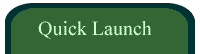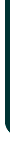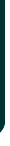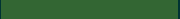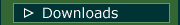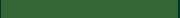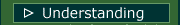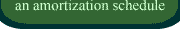VIDEOSamortizationdotcom Mortgage Calculator for iPhone

Introduction to Canadian and American Mortgages

Seminar on prepaying principal (Part A)

Seminar on prepaying principal (Part B)

Global TV Interview regarding 40 Year MortgagesLook for this logo on the Apple Store!

 < Go Back